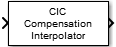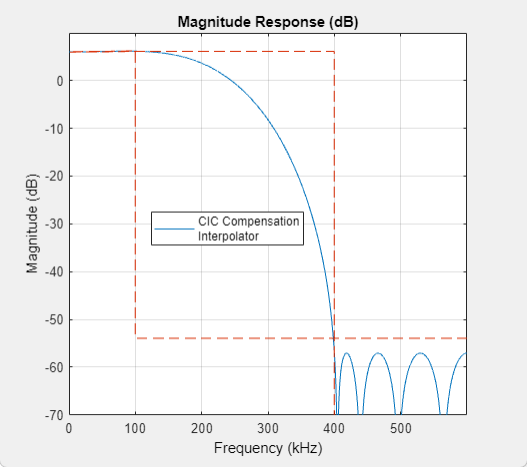# CIC Compensation Interpolator

Compensate for CIC filter using FIR interpolator

•Libraries:
DSP System Toolbox / Filtering / Multirate Filters

## Description

The CIC Compensation Interpolator block uses an FIR polyphase interpolator as the compensation filter. CIC compensation interpolators are multirate FIR filters that you cascade with CIC interpolators to mitigate the drawbacks of the CIC filters.

CIC interpolation filters are used in areas that require high interpolation. These filters are popular in ASICs and FPGAs, since they do not have any multipliers. CIC filters have two drawbacks:

• CIC filters have a magnitude response that causes a droop in the passband region. This magnitude response is:

$abs{\left(\frac{\mathrm{sin}\left(M\frac{\omega }{2}\right)}{\mathrm{sin}\left(\frac{\omega }{2}\right)}\right)}^{n}$

• M — Differential delay

• n — Number of stages

• ω — Normalized angular frequency

• CIC filters have a wide transition region.

The compensation interpolator filters have an inverse sinc passband response to correct for the CIC droop, and they have a narrow transition width.

This block brings the capabilities of the `dsp.CICCompensationInterpolator` System object™ to the Simulink® environment.

## Ports

### Input

expand all

Specify the data input as a vector or a matrix.

The block treats a P-by-Q input matrix as Q independent channels, interpolating each channel over the first dimension.

Data Types: `single` | `double` | `int8` | `int16` | `int32` | `int64` | `fixed point`

### Output

expand all

Upsampled and filtered signal, returned as a vector or matrix. For a P-by-Q input matrix, the output is a LP-by-Q matrix, where L is the interpolation factor.

When the output is fixed-point, it is signed only.

Data Types: `single` | `double` | `int8` | `int16` | `int32` | `int64` | `fixed point`

## Parameters

expand all

### Main Tab

Specify the rate-change factor of the CIC filter as a positive integer. The block compensates the CIC filter using an FIR polyphase interpolator as the compensation filter.

Specify the number of sections in the CIC filter as a positive integer. The block compensates the CIC filter using an FIR polyphase interpolator as the compensation filter.

Specify the differential delay value used in each comb section of the CIC filter as a positive integer. The block compensates the CIC filter using an FIR polyphase interpolator as the compensation filter.

Specify the interpolation factor L of the compensation filter as a positive integer.

When you select this check box, the block designs filter with the minimum order that meets the passband frequency, stopband frequency, passband ripple, and stopband attenuation specifications. When you clear this check box, the block designs filter with the order that you specify in the Filter order parameter.

Specify the order of the compensator filter as a positive integer.

#### Dependencies

To enable this parameter, clear the Minimum order filter design parameter.

Specify the passband edge frequency of the compensation filter as a positive real scalar in Hz. Passband edge frequency (Hz) must be less than Fs/2, where Fs is the output sample rate.

Specify the stopband edge frequency of the compensation filter as a positive scalar in Hz. Stopband edge frequency (Hz) must be less than Fs/2, where Fs is the sample rate of the output signal.

Specify the filter passband ripple of the compensation filter as a positive scalar in dB.

Specify the stopband attenuation of the compensation filter as a positive scalar in dB.

When you select this check box, the block inherits its sample rate from the input signal. When you clear this check box, you must specify the sample rate in Input sample rate (Hz).

Specify the sample rate of input signal as a positive scalar in Hz.

#### Dependencies

To enable this parameter, clear the Inherit sample rate from input parameter.

Click this button to open the Filter Visualization Tool (FVTool) and display the magnitude and phase response of the CIC Compensation Interpolator. The response is based on the values you specify in the block parameters dialog box. Changes made to these parameters update FVTool.To update the magnitude response while FVTool is running, modify the dialog box parameters and click .

Type of simulation to run. You can set this parameter to:

• `Code generation` (default)

Simulate model using generated C code. The first time you run a simulation, Simulink generates C code for the block. The C code is reused for subsequent simulations, as long as the model does not change. This option requires additional startup time but provides faster simulation speed than ```Interpreted execution```.

• `Interpreted execution`

Simulate model using the MATLAB®  interpreter. This option shortens startup time but has slower simulation speed than ```Code generation```.

### Data Types Tab

Select the rounding mode for fixed-point operations. The default is `Floor`.

Specify the fixed-point data type of the coefficients as one of the following:

• `fixdt(1,16)` — Signed fixed-point data type of word length `16` with binary point scaling. The block determines the fraction length automatically from the coefficient values in such a way that the coefficients occupy maximum representable range without overflowing.

• `fixdt(1,16,0)` — Signed fixed-point data type of word length `16` with fraction length `0`. You can change the fraction length to any other integer value.

• `<data type expression>` — Specify the coefficients data type by using an expression that evaluates to a data type object. For example, `numerictype`(`fixdt`(```[ ]```,`16`, `15`)). Specify the sign mode of this data type as `[ ]` or true.

• `Refresh Data Type` — Refresh to the default data type.

Click the buttonto display the data type assistant, which helps you set the coefficients data type.

## Block Characteristics

 Data Types `double` | `fixed point` | `integer` | `single` Direct Feedthrough `no` Multidimensional Signals `no` Variable-Size Signals `no` Zero-Crossing Detection `no`

## Algorithms

The response of a CIC filter is given by:

`${H}_{cic}\left(\omega \right)={\left[\frac{\mathrm{sin}\left(\frac{RD\omega }{2}\right)}{\mathrm{sin}\left(\frac{\omega }{2}\right)}\right]}^{N}$`

R, D, and N are the rate change factor, the differential delay, and the number of sections in the CIC filter, respectively.

After decimation, the CIC response has the form:

`${H}_{cic}\left(\omega \right)={\left[\frac{\mathrm{sin}\left(\frac{D\omega }{2}\right)}{\mathrm{sin}\left(\frac{\omega }{2R}\right)}\right]}^{N}$`

The normalized version of this last response is the one that the CIC compensator needs to compensate. Hence, the passband response of the CIC compensator should take the following form:

`${H}_{ciccomp}\left(\omega \right)={\left[RD\frac{\mathrm{sin}\left(\frac{\omega }{2R}\right)}{\mathrm{sin}\left(\frac{D\omega }{2}\right)}\right]}^{N}\text{for}\text{\hspace{0.17em}}\omega \le {\omega }_{p}<\pi$`

where ωp is the passband frequency of the CIC compensation filter.

Notice that when ω/2R ≪ π, the previous equation for Hciccomp(ω) can be simplified using the fact that sin(x) ≅ x:

This previous equation is the inverse sinc approximation to the true inverse passband response of the CIC filter.

## Version History

Introduced in R2015b